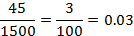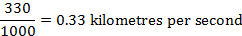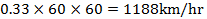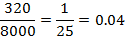# Paper 1 Project Maths Essential Revision NotesNumber Theory 3

1. Conversions. 3

2. Rounding Off 4

3. Average Speed and Fuel Consumption 7

4. Number Line 11

5. Indices and Surds 14

6. Reciprocals and Scientific Notation 19

7. Sets and Venn Diagrams 22

8. Multiples and Divisors and Prime Numbers 31

9. Percentages 33

10. Ratios 38

11. Investments and Compound Interest 44

12. Tax Credits 49

13. Exchange Rates 53

14. Factorisation 56

15. Simplifying 59

17. Division of x 67

18. Simultaneous Equations 71

19. Inequalities 77

20. Functions and Graphing 80

21. Area 90

# Conversions.

First let’s take a look at some simple conversions.

1kg=1000grams

1litre=1000ml

1metre=100centimetres

1km=1000metres=100000centimetres

1minute=60seconds

1hour=60minute=3600seconds

1.1

Express 45 centimetres as a fraction of 15 metres and write your answer in its simplest form.

Express everything in centimetres

15metres= 1500centimetres1.2

Given that the speed of sound in air is 330 metres per second, express this speed in km/hr

Change metres to kilometres and then seconds to hours1.3

Express 320 grams as a fraction of 8kg# Rounding Off

Firstly it’s important to remember in what order we multiply, add, subtract, etc…

The order is

1. Brackets

2. Exponents ( Roots and Powers)

3. Multiply or Divide

Some people like to remember the word BEMDAS to help remember the order

(Brackets Exponents Multiply Divide Add Subtract)

When rounding correct to the nearest whole number, we pay attention to the first decimal place. If it is 5 or greater we round it up to the nearest whole number. If it is less than 5 we round it down to the nearest whole number.

Look at the first decimal place

6.8 8 is greater than 5. Round up to 7.0

9.2 2 is less than 5. Round down to 9.0

5.5 If 5 is in the first decimal place we round up. Round up to 6.0

When rounding correct to one decimal place. We pay attention to the second decimal place

7.72 2 is less than 5. Round down to 7.70

16.89 9 is greater than 5. Round up to ...